Next: What is the Minkowski-Weyl Up: Convex Polyhedron Previous: What is the best   Contents

## What is convex hull? What is the convex hull problem?

For a subset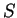of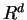, the convex hull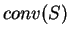is defined as the smallest convex set incontaining.

The convex hull computation means the determination'' offor a given finite set of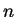points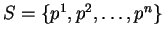in.

The usual way to determineis to represent it as the intersection of halfspaces, or more precisely, as a set of solutions to a minimal system of linear inequalities. This amounts to output a matrix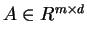and a vector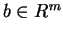for somesuch that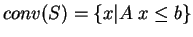. Whenis full-dimensional, each (nonredundant) inequality corresponds to a facet of. Thus the convex hull problem is also known as the facet enumeration problem, see Section 2.12.

Some people define the convex hull computation as the determination of extreme points of, or equivalently that of redundant points into determine. This is much simpler computation than our convex hull problem. In fact, this can be done by solving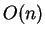linear programs and thus polynomially solvable, see Section 2.19 and 2.20. It is better to name this as the redundancy removal for a point set''.Next: What is the Minkowski-Weyl Up: Convex Polyhedron Previous: What is the best   Contents
Komei Fukuda 2004-08-26